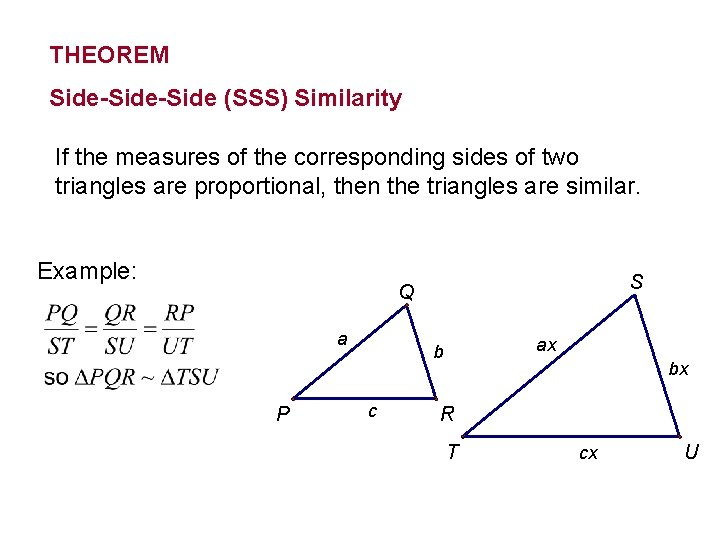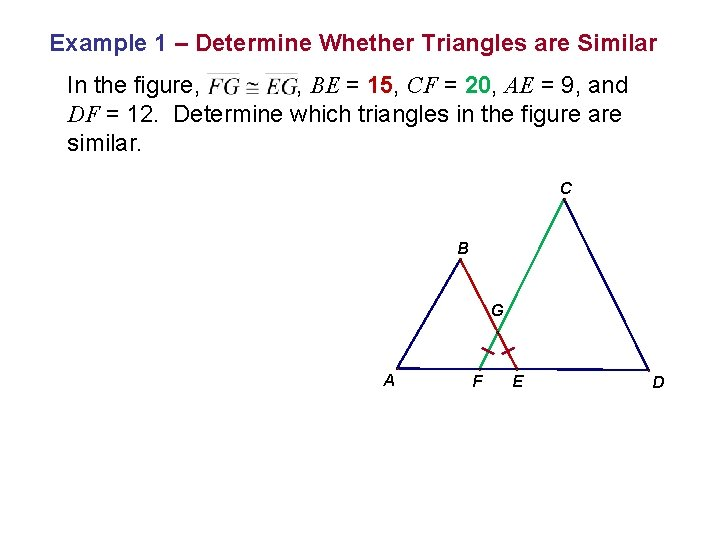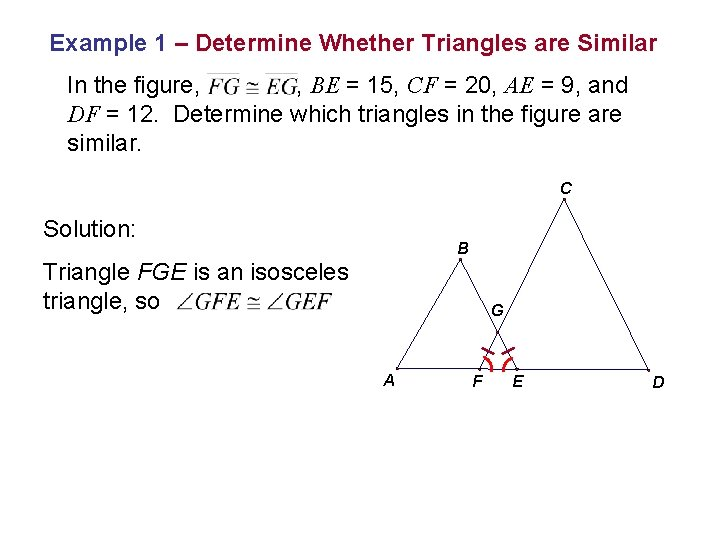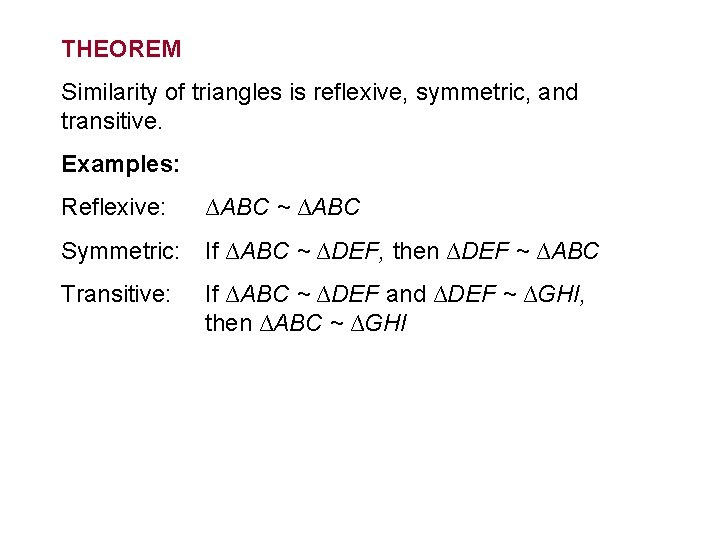SIMILAR TRIANGLES Identify similar triangles Use similar triangles

• Slides: 9SIMILAR TRIANGLES • Identify similar triangles • Use similar triangles to solve problems Eiffel TowerPOSTULATE Angle-Angle (AA) Similarity If the two angles of one triangle are congruent to two angles of another triangle, then the triangles are similar. Example:THEOREM Side-Side (SSS) Similarity If the measures of the corresponding sides of two triangles are proportional, then the triangles are similar. Example: S Q a P ax b c bx R T cx UTHEOREM Side-Angle-Side (SAS) Similarity If the measures of two sides of a triangle are proportional to the measures of two corresponding sides of another triangle and the included angles are congruent, then the triangles are similar. S Q Example: a P ax b bx R T UExample 1 – Determine Whether Triangles are Similar In the figure, , BE = 15, CF = 20, AE = 9, and DF = 12. Determine which triangles in the figure are similar. C B G A F E DExample 1 – Determine Whether Triangles are Similar In the figure, , BE = 15, CF = 20, AE = 9, and DF = 12. Determine which triangles in the figure are similar. C Solution: B Triangle FGE is an isosceles triangle, so G A F E DExample 1 – Determine Whether Triangles are Similar In the figure, , BE = 15, CF = 20, AE = 9, and DF = 12. Determine which triangles in the figure are similar. C If the measures of the corresponding sides that include the angles are proportional, then the triangles are similar. B G A By SAS similarity, ∆ABE ~ ∆DCF F E DTHEOREM Similarity of triangles is reflexive, symmetric, and transitive. Examples: Reflexive: ∆ABC ~ ∆ABC Symmetric: If ∆ABC ~ ∆DEF, then ∆DEF ~ ∆ABC Transitive: If ∆ABC ~ ∆DEF and ∆DEF ~ ∆GHI, then ∆ABC ~ ∆GHIExample 2 – Parts of Similar Triangles Algebra: Find AE and DE C A X-1 2 B 5 E X+5 D